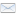Under the patronage of:Journal Metrics

CiteScore (2020): 3.7

Source Normalized Impact per Paper (SNIP): 0.589

SCImago Journal Rank (SJR): 0.384

Comparison of Analytical and Numerical Models of Adsorber/desorber of Silica Gel-water Adsorption Heat Pump

Original scientific paper

Journal of Sustainable Development of Energy, Water and Environment Systems
Volume 5, Issue 1, pp 69-88
Katarzyna Zwarycz-Makles1, Wladyslaw Szaflik2
1 West Pomeranian University of Technology, Szczecin, Szczecin, Poland
2 West Pomeranian University of Technology, Szczecin, Poland

Abstract

In the paper, comparison of an analytical and a numerical model of silica gel/water adsorber/desorber is presented. Adsorber/desorber as a part of the two-bed single-stage adsorption heat pump is discussed. The adsorption heat pump under consideration consists of an evaporator, two adsorber/desorber columns and a condenser. Only heat and mass transfer was taken into account during operation of assumed adsorption heat pump. Adsorption equilibrium was described by the Dubinin-Astachov model. The presented mathematical models, both analytical and numerical, were created to describe the temperature, heat and concentration changes in the adsorber/desorber and consequently to describe the performance of the adsorption heat pump. Heat and mass transfer equations of the model were solved analytically and calculation parameters were entered in the common spreadsheet. The numerical model was established comprising of the set of heat and mass balance partial differential equations, together with the initial and boundary conditions and was solved by the numerical method of lines. The developed analytical model is very basic and can be used only for the initial estimates of mean cyclic temperature of the cooling/heating water in the adsorber/desorber bed. Validation of the numerical model shows reasonable agreement between the numerical and experimental averaged bed temperatures and is an indication of the proper mathematical modeling and the accuracy of the numerical analysis.

Keywords: Adsorption heat pump, Silica gel adsorbent, Adsorption modeling.Views (in 2021): 306 | Downloads (in 2021): 160
Total views: 3188 | Total downloads: 2606

DBG